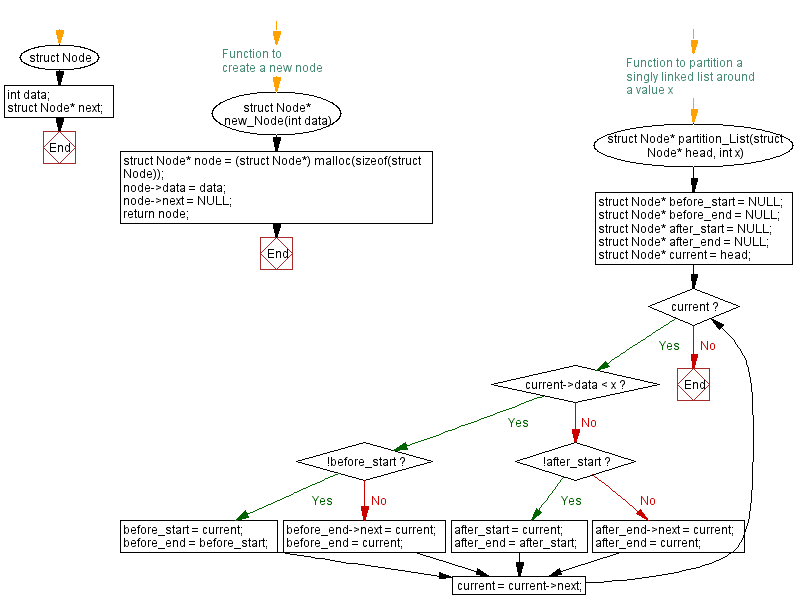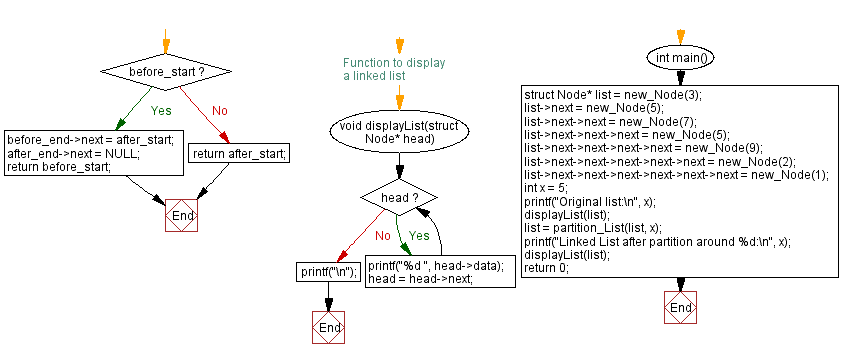﻿ C Program: Partition a singly linked list around a specific value

# C Exercises: Partition a singly linked list around a specific value

## C Singly Linked List : Exercise-21 with Solution

Write a C program to partition a singly linked list based on a specific value.

Sample Solution:

C Code:

``````#include<stdio.h>
#include <stdlib.h>

struct Node {
int data;
struct Node* next;
};

// Function to create a new node
struct Node* new_Node(int data) {
struct Node* node = (struct Node*) malloc(sizeof(struct Node));
node->data = data;
node->next = NULL;
return node;
}

// Function to partition a singly linked list around a value x
struct Node* partition_List(struct Node* head, int x) {
struct Node* before_start = NULL;
struct Node* before_end = NULL;
struct Node* after_start = NULL;
struct Node* after_end = NULL;

while (current) {
if (current->data < x) {
if (!before_start) {
before_start = current;
before_end = before_start;
} else {
before_end->next = current;
before_end = current;
}
} else {
if (!after_start) {
after_start = current;
after_end = after_start;
} else {
after_end->next = current;
after_end = current;
}
}
current = current->next;
}

if (before_start) {
before_end->next = after_start;
after_end->next = NULL;
return before_start;
} else {
return after_start;
}
}

// Function to display a linked list
}
printf("\n");
}

int main() {
struct Node* list = new_Node(3);
list->next = new_Node(5);
list->next->next = new_Node(7);
list->next->next->next = new_Node(5);
list->next->next->next->next = new_Node(9);
list->next->next->next->next->next = new_Node(2);
list->next->next->next->next->next->next = new_Node(1);

int x = 5;
printf("Original list:\n", x);
displayList(list);

list = partition_List(list, x);
printf("Linked List after partition around %d:\n", x);
displayList(list);
return 0;
}
```
```

Sample Output:

```Original list:
3 5 7 5 9 2 1
Linked List after partition around 5:
3 2 1 5 7 5 9
```

Flowchart :C Programming Code Editor:

What is the difficulty level of this exercise?

﻿